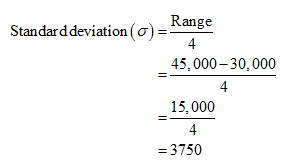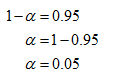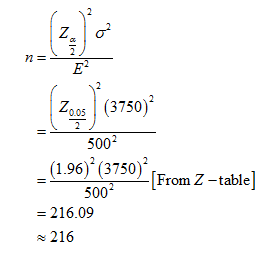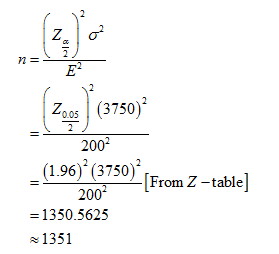# Annual starting salaries for college graduates with degrees in business administration are generally expected to be between \$30,000 and \$45,000. Assume that a 95% confidence interval estimate of the population mean annual starting salary is desired. a. What is the planning value for the population standard deviation? How large a sample should be taken if the desired margin of error isb.\$500?c.\$200?d.\$100?e.Would you recommend trying to obtain the \$100 margin of error? Explain.

Question
23 views

Annual starting salaries for college graduates with degrees in
\$45,000. Assume that a 95% confidence interval estimate of the population mean annual starting salary is desired.

a. What is the planning value for the population standard deviation?

How large a sample should be taken if the desired margin of error is
b.\$500?

c.\$200?

d.\$100?

e.Would you recommend trying to obtain the \$100 margin of error? Explain.

check_circle

star
star
star
star
star
1 Rating
Step 1

a.

From the given information, annual starting salaries are expected to be between \$30,000 and \$45,000.

Then, the planning value for the population standard deviation isThus, the planning value for the population standard deviation is \$3,750.

b.

From the given information,Then, the sample size for the margin of error \$500 isThus, the sample size for the margin of error \$500 is 216.

Step 2

c.

The sample size for the margin of error \$200 isThus, the sample size for the margin of error \$200 is 1351.

...

### Want to see the full answer?

See Solution

#### Want to see this answer and more?

Solutions are written by subject experts who are available 24/7. Questions are typically answered within 1 hour.*

See Solution
*Response times may vary by subject and question.
Tagged in